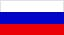ISSN 2079-3537

Scientific Visualization, 2019, volume 11, number 2, pages 156 - 162, DOI: 10.26583/sv.11.2.12

On visualization problems in a generalized computational experiment

Author: A.E. Bondarev 1

Keldysh Institute of Applied Mathematics RAS

1 ORCID: 0000-0003-3681-5212, bond@keldysh.ru

Abstract

Modern development of computing systems and technologies allows to organize the construction of a generalized computational experiment in modeling problems of computational gas dynamics. The construction of such an experiment is based on numerical parametric studies and the solution of optimization analysis problems. Solving such problems implies a multiple solution to the direct problem of numerical simulation of a gas-dynamic process with different input data, where different sets of defining parameters are used as input data. The defining parameters of a class of problems, such as Mach number, Reynolds number, geometric parameters, etc., vary in certain ranges with a certain partitioning step. As a result, the resulting solution is a multidimensional volume of data. To analyze this volume, visualization is necessary. The paper attempts to systematize and briefly describe some types of visualization problems arising from processing the results of a generalized computational experiment. The aim of the work is to begin a broad discussion of the tasks of visualization and methods for their solution in a generalized computational experiment.

Keywords: generalized computational experiment, CFD, multidimensional data, visualization, visual analysis.